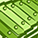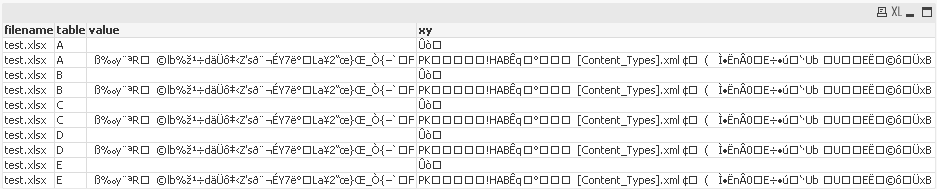# QlikView App Dev

Discussion Board for collaboration related to QlikView App Development.

Announcements
Skip the ticket, Chat with Qlik Support instead for instant assistance.
cancel
Showing results for
Did you mean:Anonymous
Not applicable

Hi,

I have the following script to load in numerous tab from an excel spreadsheet. I only need to use row 1 and 2 and then transpose them. All tabs have the same format, it simply that some of them contain data in row 2 and others do not.

I am getting an error "Field not found - <@2>" when there is no data in row 2 of some of the excel tabs. What is best way to get around this error.

for each vSheet in 'A', 'B', 'C',...........etc

Initial_Current_Data:

@1 as xy,

@2 as value,

'\$(vSheet)' as table,

FileName() as filename

FROM

[\$(Location)]

(ooxml, no labels, table is \$(vSheet), filters(

Transpose()

));

next;

1 Solution

Accepted SolutionsChampion

Check this script.

Set vFilePath = 'C:\Users\918271\Desktop\test.xlsx';

for each vSheet in 'A', 'B', 'C', 'D', 'E'

RowCount:
FROM
'\$(vFilePath)'
(
ooxml, no labels, table is \$(vSheet)) Where RecNo()=2;

Let vRowCount = Peek('A',0,'RowCount');
DROP Table RowCount;

If Len(Trim('\$(vRowCount)'))>0 THEN

Initial_Current_Data:
@2 as value
'\$(vSheet)'
as table,
FileName() as filename
FROM
'\$(vFilePath)'
(
ooxml, no labels, table is \$(vSheet), filters(
Transpose()
));

ENDIF

next;

11 RepliesSpecialist II

i tried to reconstruct your problem. For me it will load it without error even if there are no data.

you can try

1) if(len(trim(@2))=0,  null(), @2) as value

but i dont think that this will work

2) can try to set error mode to 0

SET ErrorMode = 0;

SET ErrorMode = 1

i dont know if this ignores the error or simply skips the failed load statement. try itAnonymous
Not applicable
Author

Thanks for this Tim.

Option 1 does not work as it is still calling out the "field" @2 which does not exist. I had tried this error.

Option 2 does work but I am worried that I will miss errors in my script - its a long one - by taking this route.

Do you happen to know how to count the number of rows per table (i.e. for the loop) and then load only those tables that have a row count of over greater than 1?Specialist IIChampion

Hi Hasham,

I have also tried to replicate the problem but it is working fine. Could you attach a sample file.Anonymous
Not applicable
Author

for each vSheet in 'A', 'B', 'C', 'D', 'E'

Initial_Current_Data:

@2 as value,

'\$(vSheet)' as table,

FileName() as filename

FROM

(ooxml, no labels, table is \$(vSheet), filters(

Transpose()

));

next;Specialist II

you can also try to Change your Format

from

(ooxml)

to

(txt)Champion

Check this script.

Set vFilePath = 'C:\Users\918271\Desktop\test.xlsx';

for each vSheet in 'A', 'B', 'C', 'D', 'E'

RowCount:
FROM
'\$(vFilePath)'
(
ooxml, no labels, table is \$(vSheet)) Where RecNo()=2;

Let vRowCount = Peek('A',0,'RowCount');
DROP Table RowCount;

If Len(Trim('\$(vRowCount)'))>0 THEN

Initial_Current_Data:
@2 as value
'\$(vSheet)'
as table,
FileName() as filename
FROM
'\$(vFilePath)'
(
ooxml, no labels, table is \$(vSheet), filters(
Transpose()
));

ENDIF

next;Anonymous
Not applicable
Author

thanks Tim - no error but the output is now incorrect.Anonymous
Not applicable
Author

this work perfectly. thank you so much.Community Browser